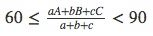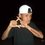# In a given triangle ABC, a=BC, b=AC, and c=AB where ABC are angle measurements of the triangle. Can you prove that the following inequality is true? Moreover, is there any maximum value of the fraction in the center of the inequality?In a given triangle ABC, a=BC, b=AC, and c=AB where ABC are angle measurements of the triangle. Can you prove that the following inequality is true? Moreover, is there any maximum value of the fraction in the center of the inequality?Note by Kayu Kaou
6 years, 9 months ago

This discussion board is a place to discuss our Daily Challenges and the math and science related to those challenges. Explanations are more than just a solution — they should explain the steps and thinking strategies that you used to obtain the solution. Comments should further the discussion of math and science.

When posting on Brilliant:

• Use the emojis to react to an explanation, whether you're congratulating a job well done , or just really confused .
• Ask specific questions about the challenge or the steps in somebody's explanation. Well-posed questions can add a lot to the discussion, but posting "I don't understand!" doesn't help anyone.
• Try to contribute something new to the discussion, whether it is an extension, generalization or other idea related to the challenge.

MarkdownAppears as
*italics* or _italics_ italics
**bold** or __bold__ bold
- bulleted- list
• bulleted
• list
1. numbered2. list
1. numbered
2. list
Note: you must add a full line of space before and after lists for them to show up correctly
paragraph 1paragraph 2

paragraph 1

paragraph 2

[example link](https://brilliant.org)example link
> This is a quote
This is a quote
    # I indented these lines
# 4 spaces, and now they show
# up as a code block.

print "hello world"
# I indented these lines
# 4 spaces, and now they show
# up as a code block.

print "hello world"
MathAppears as
Remember to wrap math in $$ ... $$ or $ ... $ to ensure proper formatting.
2 \times 3 $2 \times 3$
2^{34} $2^{34}$
a_{i-1} $a_{i-1}$
\frac{2}{3} $\frac{2}{3}$
\sqrt{2} $\sqrt{2}$
\sum_{i=1}^3 $\sum_{i=1}^3$
\sin \theta $\sin \theta$
\boxed{123} $\boxed{123}$

Sort by:

INDONESIA, makassar. abdui,karim

- 6 years, 9 months ago

This week, we continue our study of Group Theory with a guest post by Chu-Wee Lim on Lagrange's Theorem.

H=4

- 6 years, 9 months ago

Brilliant user Tan asks if it is possible to convert a 4-dimension space to a 3-dimension space in the same way we can convert our 3-dimension world into a 2-dimension map.

6 box

- 6 years, 9 months ago

Determine the smallest integer that is divisible by 24 and has digit sum equal to 24.

- 6 years, 9 months ago

Since 24 = 8 * 3, the sum of the digits must be = 0 (mod 3), which is satisfied by the fact that the sum of the digits must be 24. Therefore, we ignore this condition, so now we need the smallest n = 0 (mod 8 ), whose sum of digits = 24. We clearly

- 6 years, 9 months ago

Brilliant's physics problems this week are all about the hyperloop, a new high-speed transportation system proposed by inventor Elon Musk. The hyperloop is a tube through which capsules move at a top speed of 1,220 kilometers per hour (760 miles per hour). Our problems explore some of the technical challenges it faces as well as the benefits it provides.

- 6 years, 9 months ago

6v1 4v1 2v1 2v2 4v1 2v0

- 6 years, 9 months ago

6 1 _+ 6

4 1 __+ 5

2 1 _+ 3

2 2 __+ 4

4 1 __+ 5

2 0 __+ 20

- 6 years, 9 months ago